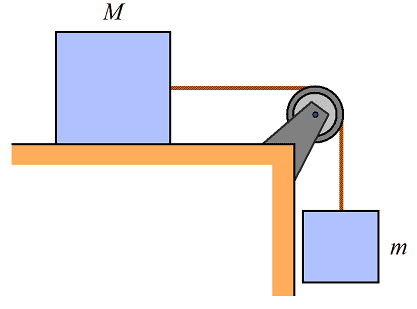# Static Friction Question — A wood block sliding on a tabletop

• physicsstudent111

#### physicsstudent111

Homework Statement
A 4.0 kg block of wood (m 1 ) sits on a level table. A string is tied to the wood and runs over a
frictionless pulley to a hanging object (as shown below). The greatest mass that can be hung
from the string (m 2 ) without moving the block of wood is 1.8 kg. Calculate the coefficient of
static frictions between the block of wood and the table.
Relevant Equations
F=uFn
Fg=Fn
I have no idea how to start this other than that I think that Fn is 17.64N and Fnet is 0. But I don't have the mew, so I am not sure how I am going to get the force of static friction.

Welcome!

About a week. Do you have any suggestions for this question?

About a week. Do you have any suggestions for this question?
Draw a free body diagram (FBD) for the block. They are the cat's mew for problems of this kind.

•bob012345, berkeman and Lnewqban
What is Fn in that equation?
Show us how did you estimate that value of 17.64 N, please.

I assumed that Force of gravity is equal to Fn. So I did 1.8kg(9.80m/s(down))=17.64N. But I think this is wrong

Yes, you have calculated the weight of block #2, but that one is hanging in the air (no friction there).•jbriggs444